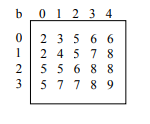﻿ Java Search Exercises - w3resource# Java Search: Exercises, Practice, Solution

## Java Search Exercises [7 exercises with solution]

[An editor is available at the bottom of the page to write and execute the scripts.]

1. Write a Java program to find a specified element in a given array of elements using Binary Search. Go to the editor
Click me to see the solution

2. Write a Java program to find a specified element in a given array of elements using Linear Search. Go to the editor
Click me to see the solution

3. Write a Java program to find a specified element in a given sorted array of elements using Jump Search. Go to the editor
From Wikipedia, in computer science, a jump search or block search refers to a search algorithm for ordered lists. It works by first checking all items Lkm, where ℜ ∈ ℵ and m is the block size, until an item is found that is larger than the search key. To find the exact position of the search key in the list a linear search is performed on the sublist L[(k-1)m, km].
Click me to see the solution

4. Write a Java program to find a specified element in a given array of elements using Interpolation Search. Go to the editor
From Wikipedia, Interpolation search is an algorithm for searching for a key in an array that has been ordered by numerical values assigned to the keys (key values). It was first described by W. W. Peterson in 1957. Interpolation search resembles the method by which people search a telephone directory for a name (the key value by which the book's entries are ordered): in each step the algorithm calculates where in the remaining search space the sought item might be, based on the key values at the bounds of the search space and the value of the sought key, usually via a linear interpolation. The key value actually found at this estimated position is then compared to the key value being sought. If it is not equal, then depending on the comparison, the remaining search space is reduced to the part before or after the estimated position. This method will only work if calculations on the size of differences between key values are sensible.
Click me to see the solution

5. Write a Java program to find a specified element in a given sorted array of elements using Exponential search. Go to the editor

From Wikipedia, in computer science, an exponential search (also called doubling search or galloping search or Struzik search) is an algorithm, created by Jon Bentley and Andrew Chi-Chih Yao in 1976, for searching sorted, unbounded/infinite lists. There are numerous ways to implement this with the most common being to determine a range that the search key resides in and performing a binary search within that range. This takes O(log i) where i is the position of the search key in the list, if the search key is in the list, or the position where the search key should be, if the search key is not in the list.
Click me to see the solution

6. Write a Java program to find a specified element in a given array of elements using Ternary search. Go to the editor

From Wikipedia, a ternary search algorithm is a technique in computer science for finding the minimum or maximum of a unimodal function. A ternary search determines either that the minimum or maximum cannot be in the first third of the domain or that it cannot be in the last third of the domain, then repeats on the remaining two thirds. A ternary search is an example of a divide and conquer algorithm.
Click me to see the solution

7. Given is a 2-dimensional integer array [0..m-1, 0..n-1], each row and column of which is in ascending order (see example)Write a Java program to find the row, column position of a specified number (row, column position) in a given 2-dimensional array. Go to the editor
Click me to see the solution

Java Code Editor:

More to Come !

Do not submit any solution of the above exercises at here, if you want to contribute go to the appropriate exercise page.

﻿

## Java: Tips of the Day

Why is (a*b != 0) faster than (a != 0 && b != 0) in Java?

```if (a != 0 && b != 0)
```

will compile to 2 memory loads and two conditional branches

```if (a * b != 0)
```

will compile to 2 memory loads, a multiply and one conditional branch.

The multiply is likely to be faster than the second conditional branch if the hardware-level branch prediction is ineffective. As you increase the ratio ... the branch prediction is becoming less effective.

The reason that conditional branches are slower is that they cause the instruction execution pipeline to stall. Branch prediction is about avoiding the stall by predicting which way the branch is going to go and speculatively choosing the next instruction based on that. If the prediction fails, there is a delay while the instruction for the other direction is loaded.

Ref: https://bit.ly/3ftdl2g